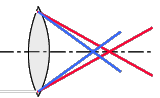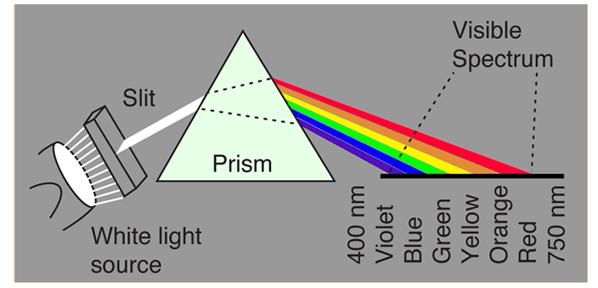# Dispersion

Chromatic dispersion is the change of index of refraction with wavelength. Generally the index decreases as wavelength increases, blue light traveling more slowly in the material than red light. Dispersion is the phenomenon which gives you the separation of colors in a prism. It also gives the generally undesirable chromatic aberration in lenses. Usually the dispersion of a material is characterized by measuring the index at the blue F line of hydrogen (486.1 nm), the yellow sodium D lines (589.3 nm), and the red hydrogen C line (656.3 nm). The dispersion is measured by a standard parameter known as Abbe's number, or the v value or V number, all of which refer to the same parameter:Data from Serway & Jewett

Blue light travels more slowly than red light in transparent media.

The effect of dispersion on the focal length of a lens can be examined by calculating the change in the focal length with wavelength. The table below starts with a biconvex lens designed to have a focal length of 10.0 cm for violet light (400 nm) in crown glass. The focal lengths shown are calculated from the lensmakers equation with radii of curvature 10.62 cm for both surfaces.

 Medium Violet400 nm Red650 nm Crown glass 10.00 10.37 Acrylic 10.46 10.87 Fused quartz 11.30 11.58Chromatic aberration arising from dispersion.Separation of colors by a prism is an example of dispersion.
 Example of dispersion calculation
Index

Lens concepts

Reference
Serway & Jewett

 HyperPhysics***** Light and Vision R Nave
Go Back

# Example of Dispersion

Dispersion in optical glasses is typically characterized by index of refraction values at three standard wavelengths.Note that a low v value implies high dispersion.

 Example of using low and high dispersion glasses in a doublet.
Index

Lens concepts

"Reference
Meyer-Arendt
Ch 1

 HyperPhysics***** Light and Vision R Nave
Go Back